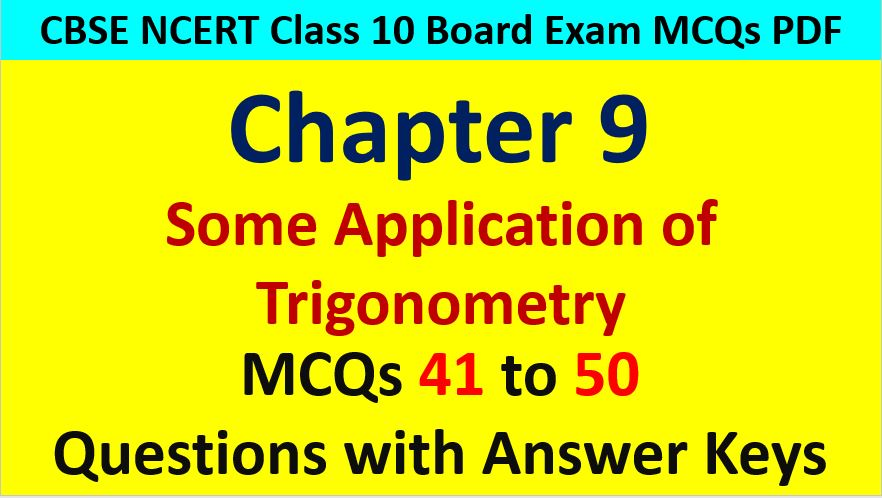Friday, October 22, 2021
Home > CBSE Class 10 > Application of Trigonometry CBSE Class 10 MCQ Questions with Answers Keys

# Application of Trigonometry CBSE Class 10 MCQ Questions with Answers KeysHi students, Welcome to AMBiPi (Amans Maths Blogs). In this article, you will get Application of Trigonometry CBSE Class 10 MCQ Questions with Answer Keys. You can download this PDF and save it in your mobile device or laptop etc.

Height & Distance CBSE Class 10 MCQ Question No 41:

Two poles, one is double in length of other, are standing opposite to each other at a distance of y meter. If the angle of elevation from the mid point of the line joining their feet are complementary then what is the height of the shorter pole?

Option A : y/√2

Option B : y/2√2

Option C : y/2

Option D : y/(√2 + 1)

Option B : y/2√2

Height & Distance CBSE Class 10 MCQ Question No 42:

The length of the tight thread of a kite from a point on the ground is 85 m. If thread subtends an angle θ with the ground such that tanθ = 8/15, then find the height at which kite is flying.

Option A : 85 m

Option B : 17 m

Option C : 40 m

Option D : 80 m

Option C : 17 m

Height & Distance CBSE Class 10 MCQ Question No 43:

In a storm, a tree got bent by the wind whose top meets the ground at an angle of 30 degree, at a distance of 30 m from the root. What is the height of the tree?

Option A : 30 m

Option B : 60 m

Option C : 30√3 m

Option D : 40√3 m

Option C : 30√3 m

Height & Distance CBSE Class 10 MCQ Question No 44:

The height of a tower is 50 m. When the Sun’s altitude increases from 30 degree to 45 degrees, the length of the shadow of the tower is decreased by x meter. Find the value of x.

Option A : 50(√3 – 1)

Option B : 50(√3 + 1)

Option C : 50√3 + 1

Option D : 50√3 – 1

Option A : 50(√3 – 1)

Height & Distance CBSE Class 10 MCQ Question No 45:

A person standing on the bank of a river observes that the angle of elevation of the top of tree standing on the opposite bank is 60 degree. When he moves 40 m away from the bank, he finds that the angle of elevation to be 30 degree. Find the width of the river (in m).

Option A : 20√3

Option B : 20

Option C : 40

Option D : 40√3

Option B : 20

Height & Distance CBSE Class 10 MCQ Question No 46:

The angle of elevation θ of the top of vertical tower from a point on the ground is tanθ where tanθ = 5/12. On walking 192 meters towards the tower in same straight line, the elevation is α where tanα = 3/4. Find the height of the tower.

Option A : 180 m

Option B : 200 m

Option C : 220 m

Option D : 200 m

Option A : 180 m

Height & Distance CBSE Class 10 MCQ Question No 47:

The angle of depression of a point on the ground from the top of a tree is 60 degree. Lowering down 20 m from the top, the angle of depression changes to 30 degrees. Find the height of the tree.

Option A : 10

Option B : 10√3

Option C : 30√3

Option D : 30

Option D : 30

Height & Distance CBSE Class 10 MCQ Question No 48:

At the foot of a mountain the elevation of its summit is 45 degree. After ascending 2000 m towards the mountain upon an incline of 30 degree, the elevation changes to 60 degree. Find the height of the mountain?

Option A : 2000(√3 – 1) m

Option B : 1000(√3 – 1) m

Option C : 2000(√3 + 1) m

Option D : 1000(√3 + 1) m

Option C : 1000(√3 – 1) m

Height & Distance CBSE Class 10 MCQ Question No 49:

From a point of the ground, the angle of elevation of a top of a tower is 30 degrees. On moving 50√3 meter towards the tower the elevation changes to 60 degree. Find the height of the tower?

Option A : 75√3 m

Option B : 75 m

Option C : 90 m

Option D : 60√3 m

Option B : 75 m

Height & Distance CBSE Class 10 MCQ Question No 50:

P and Q are two points observed from the top of a building 10√3 m high. If the angles of depression of the points are complementary and PQ = 20 m, then the distance of P from the building is

Option A : 30 m

Option B : 40 m

Option C : 25 m

Option D : 45 m